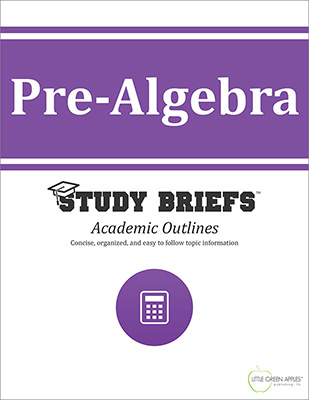# Pre-AlgebraLook inside
• Format: eBook
• Edition: 2016
• Publisher: Little Green Apples Publishing LLC

Price: \$4.99

## Description:

This Pre-Algebra Study Brief focuses on basic mathematical concepts and contains a review of foundational facts and principles needed to prepare for learning Algebra today. This academic outline offers a clear and concise conceptual overview with coherent definitions, straightforward explanations, and numerous instructive examples that are easy to follow and simple to understand. This Study Brief is intended as a quick reference for students ready to advance from general math topics into higher-levels of high school or college mathematics. It is also a convenient refresher for lifelong adult learners interested in a condensed summary of the fundamentals required to comprehend and solve basic everyday algebra problems. Sample equations and formulas are included in an organized and highly visual format. Example problems with worked-out answers serve as a useful resource and handy reference for future independent problem solving. This Study Brief emphasizes basic elements of algebra and practical methods of computation that are needed to achieve a solid foundation of comprehension before moving on to use more advanced algebraic methods. Individual sections cover whole numbers and their basic properties, divisibility rules, properties of operations, grouping and order of operations, integers, ordered pairs and relations, factors and multiples, fractions, measures of central tendency, powers and exponents, squares and square roots, negative exponents, scientific notation, metric system, geometry basics, variables, formulas, equations and inequalities.

## Topics Covered:

• Whole Numbers and Their Basic Properties
• Divisibility Rules
• Properties of Operations
• Grouping and Order of Operations
• Integers
• Ordered Pairs and Relations
• Factors and Multiples
• Fractions
• Measures of Central Tendency
• Powers and Exponents/Squares and Square Roots/Negative Exponents
• Scientific Notation
• Metric System
• Geometry Basics
• Variables
• Formulas and Equations
• Solving Two-Step Equations
• Writing Inequalities
• References

Browse Subjects